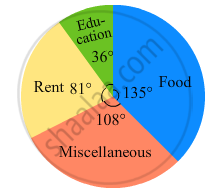Share

# The Following Pie-chart Shows the Monthly Expenditure of Shikha on Various Items. If She Spends Rs 16000 per Month, Answer the Following Questions: - Mathematics

Course
ConceptIntroduction of Circle Graph Or Pie Chart

#### Question

The following pie-chart shows the monthly expenditure of Shikha on various items. If she spends Rs 16000 per month, answer the following questions:(i) How much does she spend on rent?
(ii) How much does she spend on education?
(iii) What is the ratio of expenses on food and rent?

#### Solution

(i) Money spent on rent $= \frac{\text{ Central angle of the corresponding sector }\times\text{ Total Money spent }}{360^\circ}$
$= \frac{81^\circ \times 16000}{360^\circ} =\text{ Rs }3, 600$
(ii) Money spent on education $= \frac{\text{ Central angle of the corresponding sector }\times \text{ Total Money spent }}{360^\circ}$
$= \frac{36^\circ \times 16000}{360^\circ} =\text{ Rs }1, 600$
(iii) Money spent on food $= \frac{\text{ Central angle of the corresponding sector }\times\text{ Total Money spent }}{360^\circ}$
$= \frac{135^\circ \times 16000}{360^\circ} = 6, 000$
$\text{ Ratio of expenses on food and rent }= \frac{6000}{3600} = \frac{5}{3}$

Is there an error in this question or solution?

#### APPEARS IN

RD Sharma Solution for Mathematics for Class 8 by R D Sharma (2019-2020 Session) (2017 to Current)
Chapter 25: Data Handling-III (Pictorial Representation of Data as Pie Charts or Circle Graphs)
Ex. 25.2 | Q: 6 | Page no. 23

#### Video TutorialsVIEW ALL 

Solution The Following Pie-chart Shows the Monthly Expenditure of Shikha on Various Items. If She Spends Rs 16000 per Month, Answer the Following Questions: Concept: Introduction of Circle Graph Or Pie Chart.
S